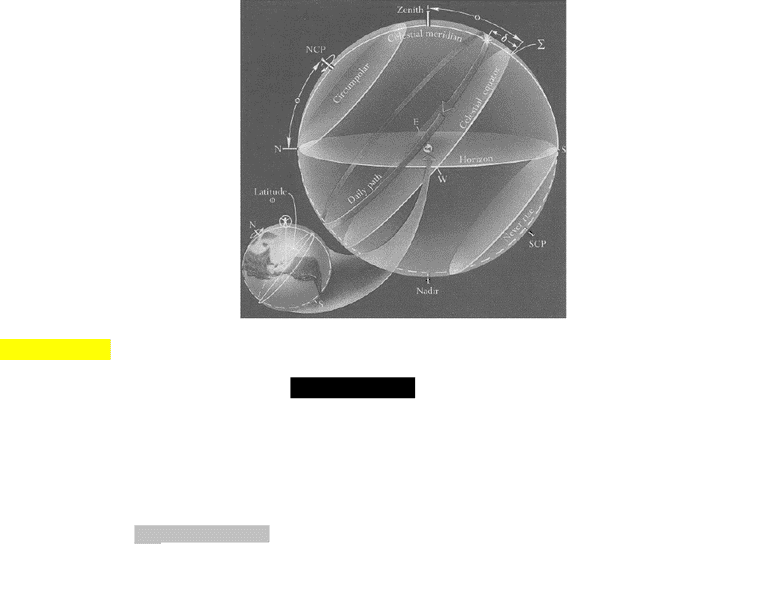Study Guides (400,000)
CA (150,000)
UTSC (10,000)
Midterm

# ASTA01H3 Study Guide - Midterm Guide: Memory Stick, Escape Velocity, Apsis

Department
Astronomy
Course Code
ASTA01H3
Professor
Parandis Tajbakhsh
Study Guide
Midterm

This preview shows pages 1-2. to view the full 6 pages of the document.ASTRONOMY MIDTERM REVIEW
LECTURE #1
-Scientific notation
-Tkelvin = Tcelsius + 273
-Astronomical measurements
o1 AU = 150, 000, 000 km
o1 ly = 63, 000 AU
Distance = speed x time
-Speed of light: 3x108 m/s
oExample. Convert 642ly into astronomical units.
642(63, 000) = 4.0x107 AU
oExample. How long does it take to get from sun to Jupiter if distance is 7.4x108 km?
t = [7.4x1011 m / 3x108 m/s] / 60
= ~40 mins
LECTURE #2
-Apparent Magnitude: the apparent brightness of a star as observed from Earth
oObserved apparent brightness depends on intrinsic brightness and distance
-Brightness
oMagnitude 1 (brightest) -------------- Magnitude 6 (faintest)
-Flux
F1/F2 = 2.5(m2-m1)
oExample. Star 1 m1= -1.5 while star 2 m2= 8. Which is brighter?
F1/F2 = 2.5(8- (-1.5))
= 39 Star 2 is 39 times brighter than star 1
LECTURE #3
-Celestial sphere
oZenith is the point right above you
oNadir is the point right below you
oNCP/SCP are points where the extension of earth’s axis pierces through the celestial sphere
oCelestial equator is an extension of the earth’s equator on the celestial sphere
oHorizon of each observer is the plane of the circle with the largest circumference perpendicular to line of
oCelestial meridian is the circle that passes through zenith, nadir, NCP/SCP
Intersection of celestial meridian and horizon defines N and S
Intersection of celestial equator and horizon defines E and W
oElevation of daily path which is parallel to the celestial equator is known as declination (δ)
- OBSERVER DEPENDENT -

Only pages 1-2 are available for preview. Some parts have been intentionally blurred.LECTURE #4
-Star rises when it comes above the horizon and sets when it’s below it
-If declination of a star is larger than 90 – latitude (θ), then that star never sets and always remains above the
horizon = CIRCUMPOLAR STAR
oExample. If Toronto’s altitude is 43.7°N, θ = 43.7
90 – 43.7 = 46.3°N
so any star 46.3°N of NCP is a circumpolar star
oExample. From Toronto, which of the following is a circumpolar star:
Sirius θ = -16°N
Betelgeus θ = 7°N
Polaris θ = 89°N
Proximas θ = -62°N
-Precession is the slow movement of the axis of a spinning body around another axis due to a torque (such as
gravitational influence) acting to change the direction of the first axis
-Ecliptic is when the sun orbits the earth which occurs once a year in a CCW motion (WE)
oSun moves on its annual path by less than 1° a day
oPlane of ecliptic is titled with respect to celestial equator
Angle between the two is OBLIQUITY OF ECLIPTIC
oIntersection of ecliptic and celestial equator is Vernal Equinox/Autumnal Equinox
At which point, declination is 0°
-Solstice is point of maximum/minimum declination
oSummer Solstice in northern hemisphere
Sun spends LONG time above horizon; rises in NE and sets in NW
oWinter Solstice in norther hemisphere
Sun spends LITTLE time above horizon; rises in SE and sets in SW